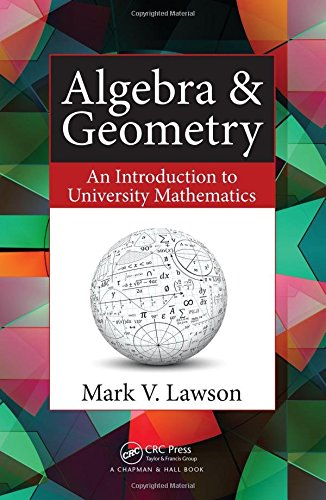ALGEBRA & GEOMETRY
An introduction to university mathematics

This is the errata page for my bookName Typos Dr James J. Ward (NUI, Galway) Page 57. Diakonis spelt here with a `k' rather than the correct `c'. Scribal error. MVL Page 25 and throughout. Illiad should be Iliad. Scribal error. Dr Roger Luther (Sussex) Page 307. There is a notation clash. In the second paragraph,  we should define d = b - a and e = c - a  (rather than c and d, since c has already been used) so that the equation of the plane then assumes the form r = a  +  $\lambda$d + $\mu$e. MVL Exercises 4.3, Question 5. Correct as far as it goes, but needs a little helping hand. For the solutions to be real we should assume in addition that a^2 -b > 0 and a > 0. If the square roots that result are to be square roots of rational numbers then a^2 - b should also be a perfect square.$a$ Amelia Wilson-Lake There is a typo in the solution to Question 4(b) of Exercises 4.4. MVL Page 299. First line of the proof of Theorem 9.3.2 should just read 'The area of the parallelogram is equal to' (delete `the absolute value of'). Lengths are, of course, non-negative! MVL Page 32. The symbol for the empty set is actually Norwegian rather than Danish and its introduction is attributed to the mathematician Andre Weil (brother of Simone Weil). Harith Faris Page 182. Proof of Lemma 7.2.3. In the displayed formula, on the RHS of the equals sign the prime should be on the first r(x) not the second.